## Search

Machine learning Filter

# What is Linear Regression in Machine Learning

9069Machine Learning, being a subset of Artificial Intelligence (AI), has been playing a dominant role in our daily lives. Data science engineers and developers working in various domains are widely using machine learning algorithms to make their tasks simpler and life easier. For example, certain machine learning algorithms enable Google Maps to find the fastest route to our destinations, allow Tesla to make driverless cars, help Amazon to generate almost 35% of their annual income, AccuWeather to get the weather forecast of 3.5 million locations weeks in advance, Facebook to automatically detect faces and suggest tags and so on.In statistics and machine learning, linear regression is one of the most popular and well understood algorithms. Most data science enthusiasts and machine learning  fanatics begin their journey with linear regression algorithms. In this article, we will look into how linear regression algorithm works and how it can be efficiently used in your machine learning projects to build better models.

Linear Regression is one of the machine learning algorithms where the result is predicted by the use of known parameters which are correlated with the output. It is used to predict values within a continuous range rather than trying to classify them into categories. The known parameters are used to make a continuous and constant slope which is used to predict the unknown or the result.

## What is a Regression Problem?

Majority of the machine learning algorithms fall under the supervised learning category. It is the process where an algorithm is used to predict a result based on the previously entered values and the results generated from them. Suppose we have an input variable ‘x’ and an output variable ‘y’ where y is a function of x (y=f{x}). Supervised learning reads the value of entered variable ‘x’ and the resulting variable ‘y’ so that it can use those results to later predict a highly accurate output data of ‘y’ from the entered value of ‘x’. A regression problem is when the resulting variable contains a real or a continuous value. It tries to draw the line of best fit from the data gathered from a number of points.For example, which of these is a regression problem?

• How much gas will I spend if I drive for 100 miles?
• What is the nationality of a person?
• What is the age of a person?
• Which is the closest planet to the Sun?

Predicting the amount of gas to be spent and the age of a person are regression problems. Predicting nationality is categorical and the closest planet to the Sun is discrete.

## What is Linear Regression?

Let’s say we have a dataset which contains information about the relationship between ‘number of hours studied’ and ‘marks obtained’. A number of students have been observed and their hours of study along with their grades are recorded. This will be our training data. Our goal is to design a model that can predict the marks if number of hours studied is provided. Using the training data, a regression line is obtained which will give minimum error. This linear equation is then used to apply for a new data. That is, if we give the number of hours studied by a student as an input, our model should be able to predict their mark with minimum error.

### Hypothesis of Linear Regression

The linear regression model can be represented by the following equation: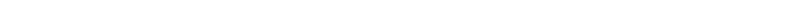where,

Y is the predicted value

θ₀ is the bias term.

θ₁,…,θn are the model parameters

x₁, x₂,…,xn are the feature values.

The above hypothesis can also be represented by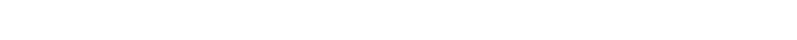Where, θ is the model’s parameter vector including the bias term θ₀; x is the feature vector with x₀ =1

Y (pred) = b0 + b1*x

The values b0 and b1 must be chosen so that the error is minimum. If sum of squared error is taken as a metric to evaluate the model, then the goal is to obtain a line that best reduces the error.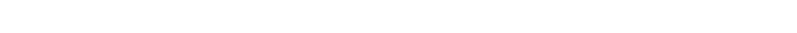If we don’t square the error, then the positive and negative points will cancel each other out.

For a model with one predictor,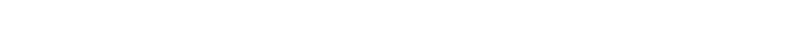Exploring ‘b1

If b1 > 0, then x (predictor) and y(target) have a positive relationship. That is an increase in x will increase y.

If b1 < 0, then x (predictor) and y(target) have a negative relationship. That is an increase in x will decrease y.

Exploring ‘b0

If the model does not include x=0, then the prediction will become meaningless with only b0. For example, we have a dataset that relates height(x) and weight(y). Taking x=0 (that is height as 0), will make the equation have only b0 value which is completely meaningless as in real-time height and weight can never be zero. This resulted due to considering the model values beyond its scope.

If the model includes value 0, then ‘b0’ will be the average of all predicted values when x=0. But, setting zero for all the predictor variables is often impossible.

The value of b0 guarantees that the residual will have mean zero. If there is no ‘b0’ term, then the regression will be forced to pass over the origin. Both the regression coefficient and prediction will be biased.

## How does Linear Regression work?

Let’s look at a scenario where linear regression might be useful: losing weight. Let us consider that there’s a connection between how many calories you take in and how much you weigh; regression analysis can help you understand that connection. Regression analysis will provide you with a relation which can be visualized into a graph in order to make predictions about your data. For example, if you’ve been putting on weight over the last few years, it can predict how much you’ll weigh in the next ten years if you continue to consume the same amount of calories and burn them at the same rate.

The goal of regression analysis is to create a trend line based on the data you have gathered. This then allows you to determine whether other factors apart from the amount of calories consumed affect your weight, such as the number of hours you sleep, work pressure, level of stress, type of exercises you do etc. Before taking into account, we need to look at these factors and attributes and determine whether there is a correlation between them. Linear Regression can then be used to draw a trend line which can then be used to confirm or deny the relationship between attributes. If the test is done over a long time duration, extensive data can be collected and the result can be evaluated more accurately. By the end of this article we will build a model which looks like the below picture i.e, determine a line which best fits the data.### How do we determine the best fit line?

The best fit line is considered to be the line for which the error between the predicted values and the observed values is minimum. It is also called the regression line and the errors are also known as residuals. The figure shown below shows the residuals. It can be visualized by the vertical lines from the observed data value to the regression line.## When to use Linear Regression?

Linear Regression’s power lies in its simplicity, which means that it can be used to solve problems across various fields. At first, the data collected from the observations need to be collected and plotted along a line. If the difference between the predicted value and the result is almost the same, we can use linear regression for the problem.

## Assumptions in linear regression

If you are planning to use linear regression for your problem then there are some assumptions you need to consider:

• The relation between the dependent and independent variables should be almost linear.
• The data is homoscedastic, meaning the variance between the results should not be too much.
• The results obtained from an observation should not be influenced by the results obtained from the previous observation.
• The residuals should be normally distributed. This assumption means that the probability density function of the residual values is normally distributed at each independent value.

You can determine whether your data meets these conditions by plotting it and then doing a bit of digging into its structure.

## Few properties of Regression Line

Here are a few features a regression line has:

• Regression passes through the mean of independent variable (x) as well as mean of the dependent variable (y).
• Regression line minimizes the sum of “Square of Residuals”. That’s why the method of Linear Regression is known as “Ordinary Least Square (OLS)”. We will discuss more in detail about Ordinary Least Square later on.
• B1 explains the change in Y with a change in x  by one unit. In other words, if we increase the value of ‘x’ it will result in a change in value of Y.

## Finding a Linear Regression line

Let’s say we want to predict ‘y’ from ‘x’ given in the following table and assume they are correlated as “y=B0+B1∗x”

xyPredicted 'y'
12Β0+B1∗1
21Β0+B1∗2
33Β0+B1∗3
46Β0+B1∗4
59Β0+B1∗5
611Β0+B1∗6
713Β0+B1∗7
815Β0+B1∗8
917Β0+B1∗9
1020Β0+B1∗10

where,

 Std. Dev. of x 3.02765 Std. Dev. of y 6.61732 Mean of x 5.5 Mean of y 9.7 Correlation between x & y 0.989938

If the Residual Sum of Square (RSS) is differentiated with respect to B0 & B1 and the results equated to zero, we get the following equation:

B1 = Correlation * (Std. Dev. of y/ Std. Dev. of x)

B0 = Mean(Y) – B1 * Mean(X)

Putting values from table 1 into the above equations,

B1 = 2.64

B0 = -2.2

Hence, the least regression equation will become –

Y = -2.2 + 2.64*x

xY - ActualY - Predicted
120.44
213.08
335.72
468.36
5911
61113.64
71316.28
81518.92
91721.56
102024.2

As there are only 10 data points, the results are not too accurate but if we see the correlation between the predicted and actual line, it has turned out to be very high; both the lines are moving almost together and here is the graph for visualizing our predicted values:### Model Performance

After the model is built, if we see that the difference in the values of the predicted and actual data is not much, it is considered to be a good model and can be used to make future predictions. The amount that we consider “not much” entirely depends on the task you want to perform and to what percentage the variation in data can be handled. Here are a few metric tools we can use to calculate error in the model-

R – Square (R2)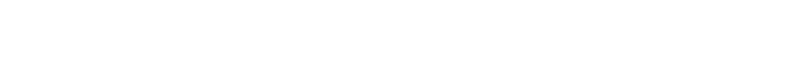Total Sum of Squares (TSS): total sum of squares (TSS) is a quantity that appears as part of a standard way of presenting results of such an analysis. Sum of squares is a measure of how a data set varies around a central number (like the mean). The Total Sum of Squares tells how much variation there is in the dependent variable.

TSS = Σ (Y – Mean[Y])2

Residual Sum of Squares (RSS): The residual sum of squares tells you how much of the dependent variable’s variation your model did not explain. It is the sum of the squared differences between the actual Y and the predicted Y.

RSS = Σ (Y – f[Y])2

(TSS – RSS) measures the amount of variability in the response that is explained by performing the regression.

#### Properties of R2

• R2 always ranges between 0 to 1.
• R2 of 0 means that there is no correlation between the dependent and the independent variable.
• R2 of 1 means the dependent variable can be predicted from the independent variable without any error.
• An R2 between 0 and 1 indicates the extent to which the dependent variable is predictable. An R2 of 0.20 means that there is 20% of the variance in Y is predictable from X; an R2 of 0.40 means that 40% is predictable; and so on.

Root Mean Square Error (RMSE)

Root Mean Square Error (RMSE) is the standard deviation of the residuals (prediction errors). The formula for calculating RMSE is: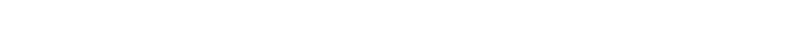Where N : Total number of observations

When standardized observations are used as RMSE inputs, there is a direct relationship with the correlation coefficient. For example, if the correlation coefficient is 1, the RMSE will be 0, because all of the points lie on the regression line (and therefore there are no errors).

Mean Absolute Percentage Error (MAPE)

There are certain limitations to the use of RMSE, so analysts prefer MAPE over RMSE which gives error in terms of percentages so that different models can be considered for the task and see how they perform. Formula for calculating MAPE can be written as: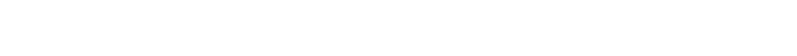Where N : Total number of observations

## Feature Selection

Feature selection is the automatic selection of attributes for your data that are most relevant to the predictive model you are working on. It seeks to reduce the number of attributes in the dataset by eliminating the features which are not required for the model construction. Feature selection does not totally eliminate an attribute which is considered for the model, rather it mutes that particular characteristic and works with the features which affects the model.

Feature selection method aids your mission to create an accurate predictive model. It helps you by choosing features that will give you as good or better accuracy whilst requiring less data. Feature selection methods can be used to identify and remove unnecessary, irrelevant and redundant attributes from the data that do not contribute to the accuracy of the model or may even decrease the accuracy of the model. Having fewer attributes is desirable because it reduces the complexity of the model, and a simpler model is easier to understand, explain and to work with.

Feature Selection Algorithms:

• Filter Method: This method involves assigning scores to individual features and ranking them. The features that have very little to almost no impact are removed from consideration while constructing the model.
• Wrapper Method: Wrapper method is quite similar to Filter method except the fact that it considers attributes in a group i.e. a number of attributes are taken and checked whether they are having an impact on the model and if not another combination is applied.
• Embedded Method: Embedded method is the best and most accurate of all the algorithms. It learns the features that affect the model while the model is being constructed and takes into consideration only those features. The most common type of embedded feature selection methods are regularization methods.

## Cost Function

Cost function helps to figure out the best possible plots which can be used to draw the line of best fit for the data points. As we want to reduce the error of the resulting value we change the process of finding out the actual result to a process which can reduce the error between the predicted value and the actual value.Here, J is the cost function.

The above function is made in this format to calculate the error difference between the predicted values and the plotted values. We take the square of the summation of all the data points and divide it by the total number of data points. This cost function J is also called the Mean Squared Error (MSE) function. Using this MSE function we are going to predict values such that the MSE value settles at the minima, reducing the cost function.

Gradient Descent is an optimization algorithm that helps machine learning models to find out paths to a minimum value using repeated steps. Gradient descent is used to minimize a function so that it gives the lowest output of that function. This function is called the Loss Function. The loss function shows us how much error is produced by the machine learning model compared to actual results. Our aim should be to lower the cost function as much as possible. One way of achieving a low cost function is by the process of gradient descent. Complexity of some equations makes it difficult to use, partial derivative of the cost function with respect to the considered parameter can provide optimal coefficient value. You may refer to the article on Gradient Descent for Machine Learning.

## Simple Linear Regression

Optimization is a big part of machine learning and almost every machine learning algorithm has an optimization technique at its core for increased efficiency. Gradient Descent is such an optimization algorithm used to find values of coefficients of a function that minimizes the cost function. Gradient Descent is best applied when the solution cannot be obtained by analytical methods (linear algebra) and must be obtained by an optimization technique.

Residual Analysis: Simple linear regression models the relationship between the magnitude of one variable and that of a second—for example, as x increases, y also increases. Or as x increases, y decreases. Correlation is another way to measure how two variables are related. The models done by simple linear regression estimate or try to predict the actual result but most often they deviate from the actual result. Residual analysis is used to calculate by how much the estimated value has deviated from the actual result.

Null Hypothesis and p-value: During feature selection, null hypothesis is used to find which attributes will not affect the result of the model. Hypothesis tests are used to test the validity of a claim that is made about a particular attribute of the model. This claim that’s on trial, in essence, is called the null hypothesis. A p-value helps to determine the significance of the results. p-value is a number between 0 and 1 and is interpreted in the following way:

• A small p-value (less than 0.05) indicates a strong evidence against the null hypothesis, so the null hypothesis is to be rejected.
• A large p-value (greater than 0.05) indicates weak evidence against the null hypothesis, so the null hypothesis is to be considered.
• p-value very close to the cut-off (equal to 0.05) is considered to be marginal (could go either way). In this case, the p-value should be provided to the readers so that they can draw their own conclusions.

## Ordinary Least Square

Ordinary Least Squares (OLS), also known as Ordinary least squares regression or least squared errors regression is a type of linear least squares method for estimating the unknown parameters in a linear regression model. OLS chooses the parameters for a linear function, the goal of which is to minimize the sum of the squares of the difference of the observed variables and the dependent variables i.e. it tries to attain a relationship between them.

There are two types of relationships that may occur: linear and curvilinear. A linear relationship is a straight line that is drawn through the central tendency of the points; whereas a curvilinear relationship is a curved line. Association between the variables are depicted by using a scatter plot. The relationship could be positive or negative, and result variation also differs in strength.

The advantage of using Ordinary Least Squares regression is that it can be easily interpreted and is highly compatible with recent computers’ built-in algorithms from linear algebra. It can be used to apply to problems with lots of independent variables which can efficiently conveyed to thousands of data points. In Linear Regression, OLS is used to estimate the unknown parameters by creating a model which will minimize the sum of the squared errors between the observed data and the predicted one.

Let us simulate some data and look at how the predicted values (Yₑ) differ from the actual value (Y):

```import pandas as pd
import numpy as np
from matplotlib import pyplot as plt

# Generate 'random' data
np.random.seed(0)
X = 2.5 * np.random.randn(100) + 1.5         # Array of 100 values with mean = 1.5, stddev = 2.5
res = 0.5 * np.random.randn(100)         # Generate 100 residual terms
y = 2 + 0.3 * X + res                    # Actual values of Y

# Create pandas dataframe to store our X and y values
df = pd.DataFrame(
{'X': X,
'y': y}
)

# Show the first five rows of our dataframe

XY
05.9101314.714615
12.5003932.076238
23.9468452.548811
37.1022334.615368
46.1688953.264107

To estimate y using the OLS method, we need to calculate xmean and ymean, the covariance of X and y (xycov), and the variance of X (xvar) before we can determine the values for alpha and beta.

```# Calculate the mean of X and y
xmean = np.mean(X)
ymean = np.mean(y)

# Calculate the terms needed for the numator and denominator of beta
df['xycov'] = (df['X'] - xmean) * (df['y'] - ymean)
df['xvar'] = (df['X'] - xmean)**2

# Calculate beta and alpha
beta = df['xycov'].sum() / df['xvar'].sum()
alpha = ymean - (beta * xmean)
print(f'alpha = {alpha}')
print(f'beta = {beta}')```
```alpha = 2.0031670124623426
beta = 0.3229396867092763```

Now that we have an estimate for alpha and beta, we can write our model as Yₑ = 2.003 + 0.323 X, and make predictions:

`ypred = alpha + beta * X`

Let’s plot our prediction ypred against the actual values of y, to get a better visual understanding of our model.

```# Plot regression against actual data
plt.figure(figsize=(12, 6))
plt.plot(X, ypred) # regression line
plt.plot(X, y, 'ro')   # scatter plot showing actual data
plt.title('Actual vs Predicted')
plt.xlabel('X')
plt.ylabel('y')

plt.show()```The blue line in the above graph is our line of best fit, Yₑ = 2.003 + 0.323 X.  If you observe the graph carefully, you will notice that there is a linear relationship between X and Y. Using this model, we can predict Y from any values of X. For example, for X = 8,

Yₑ = 2.003 + 0.323 (8) = 4.587

## Regularization

Regularization is a type of regression that is used to decrease the coefficient estimates down to zero. This helps to eliminate the data points that don’t actually represent the true properties of the model, but have appeared by random chance. The process is done by identifying the points which have deviated from the line of best-fit by a large extent. Earlier we saw that to estimate the regression coefficients β in the least squares method, we must minimize the term Residual Sum of Squares (RSS). Let the RSS equation in this case be:The general linear regression model can be expressed using a condensed formula:Here, β=[β01, ….. βp]

The RSS value will adjust the coefficient, β based on the training data. If the resulting data deviates too much from the training data, then the estimated coefficients won’t generalize well to the future data. This is where regularization comes in and shrinks or regularizes these learned estimates towards zero.

#### Ridge regression

Ridge regression is very similar to least squares, except that the Ridge coefficients are estimated by minimizing a different quantity. In particular, the Ridge regression coefficients β are the values that minimize the following quantity:Here, λ is the tuning parameter that decides how much we want to penalize the flexibility of the model. λ controls the relative impact of the two components: RSS and the penalty term. If λ = 0, the Ridge regression will produce a result similar to least squares method. If λ → ∞, all estimated coefficients tend to zero. Ridge regression produces different estimates for different values of λ. The optimal choice of λ is crucial and should be done with cross-validation. The coefficient estimates produced by ridge regression method is also known as the L2 norm.

The coefficients generated by Ordinary Least Squares method is independent of scale, which means that if each input variable is multiplied by a constant, the corresponding coefficient will be divided by the same constant, as a result of which the multiplication of the coefficient and the input variables will remain the same. The same is not true for ridge regression and we need to bring the coefficients to the same scale before we perform the process. To standardize the variables, we must subtract their means and divide it by their standard deviations.

#### Lasso Regression

Least Absolute Shrinkage and Selection Operator (LASSO) regression also shrinks the coefficients by adding a penalty to the sum of squares of the residuals, but the lasso penalty has a slightly different effect. The lasso penalty is the sum of the absolute values of the coefficient vector, which corresponds to its L1 norm. Hence, the lasso estimate is defined by:Similar to ridge regression, the input variables need to be standardized. The lasso penalty makes the solution nonlinear, and there is no closed-form expression for the coefficients as in ridge regression. Instead, the lasso solution is a quadratic programming problem and there are available efficient algorithms that compute the entire path of coefficients that result for different values of λ with the same computational cost as for ridge regression.

The lasso penalty had the effect of gradually reducing some coefficients to zero as the regularization increases. For this reason, the lasso can be used for the continuous selection of a subset of features.

## Linear Regression with multiple variables

Linear regression with multiple variables is also known as "multivariate linear regression". We now introduce notation for equations where we can have any number of input variables.

x(i)j=value of feature j in the ith training example

x(i)=the input (features) of the ith training example

m=the number of training examples

n=the number of features

The multivariable form of the hypothesis function accommodating these multiple features is as follows:

hθ(x)=θ01x12x23x3+⋯+θnxn

In order to develop intuition about this function, we can think about θ0 as the basic price of a house, θ1 as the price per square meter, θ2 as the price per floor, etc. x1 will be the number of square meters in the house, x2 the number of floors, etc.

Using the definition of matrix multiplication, our multivariable hypothesis function can be concisely represented as:This is a vectorization of our hypothesis function for one training example; see the lessons on vectorization to learn more.

Remark: Note that for convenience reasons in this course we assume x0 (i) =1 for (i∈1,…,m). This allows us to do matrix operations with θ and x. Hence making the two vectors ‘θ’and x(i) match each other element-wise (that is, have the same number of elements: n+1).

## Multiple Linear Regression

### How is it different?

In simple linear regression we use a single independent variable to predict the value of a dependent variable whereas in multiple linear regression two or more independent variables are used to predict the value of a dependent variable. The difference between the two is the number of independent variables. In both cases there is only a single dependent variable.

### Multicollinearity

Multicollinearity tells us the strength of the relationship between independent variables. Multicollinearity is a state of very high intercorrelations or inter-associations among the independent variables. It is therefore a type of disturbance in the data, and if present in the data the statistical inferences made about the data may not be reliable. VIF (Variance Inflation Factor) is used to identify the Multicollinearity. If VIF value is greater than 4, we exclude that variable from our model.

There are certain reasons why multicollinearity occurs:

• It is caused by an inaccurate use of dummy variables.
• It is caused by the inclusion of a variable which is computed from other variables in the data set.
• Multicollinearity can also result from the repetition of the same kind of variable.
• Generally occurs when the variables are highly correlated to each other.

Multicollinearity can result in several problems. These problems are as follows:

• The partial regression coefficient due to multicollinearity may not be estimated precisely. The standard errors are likely to be high.
• Multicollinearity results in a change in the signs as well as in the magnitudes of the partial regression coefficients from one sample to another sample.
• Multicollinearity makes it tedious to assess the relative importance of the independent variables in explaining the variation caused by the dependent variable.

### Iterative Models

Models should be tested and upgraded again and again for better performance. Multiple iterations allows the model to learn from its previous result and take that into consideration while performing the task again.

## Making predictions with Linear Regression

Linear Regression can be used to predict the value of an unknown variable using a known variable by the help of a straight line (also called the regression line). The prediction can only be made if it is found that there is a significant correlation between the known and the unknown variable through both a correlation coefficient and a scatterplot.

The general procedure for using regression to make good predictions is the following:

• Research the subject-area so that the model can be built based on the results produced by similar models. This research helps with the subsequent steps.
• Collect data for appropriate variables which have some correlation with the model.
• Specify and assess the regression model.
• Run repeated tests so that the model has more data to work with.

To test if the model is good enough observe whether:

• The scatter plot forms a linear pattern.
• The correlation coefficient r, has a value above 0.5 or below -0.5. A positive value indicates a positive relationship and a negative value represents a negative relationship.

If the correlation coefficient shows a strong relationship between variables but the scatter plot is not linear, the results can be misleading. Examples on how to use linear regression have been shown earlier.

## Data preparation for Linear Regression

##### Step 1: Linear Assumption

The first step for data preparation is checking for the variables which have some sort of linear correlation between the dependent and the independent variables.

##### Step 2: Remove Noise

It is the process of reducing the number of attributes in the dataset by eliminating the features which have very little to no requirement for the construction of the model.

##### Step 3: Remove Collinearity

Collinearity tells us the strength of the relationship between independent variables. If two or more variables are highly collinear, it would not make sense to keep both the variables while evaluating the model and hence we can keep one of them.

##### Step 4: Gaussian Distributions

The linear regression model will produce more reliable results if the input and output variables have a Gaussian distribution. The Gaussian theorem states that  states that a sample mean from an infinite population is approximately normal, or Gaussian, with mean the same as the underlying population, and variance equal to the population variance divided by the sample size. The approximation improves as the sample size gets large.

##### Step 5: Rescale Inputs

Linear regression model will produce more reliable predictions if the input variables are rescaled using standardization or normalization.

## Linear Regression with statsmodels

We have already discussed OLS method, now we will move on and see how to use the OLS method in the statsmodels library. For this we will be using the popular advertising dataset. Here, we will only be looking at the TV variable and explore whether spending on TV advertising can predict the number of sales for the product. Let’s start by importing this csv file as a pandas dataframe using read_csv():

```# Import and display first five rows of advertising dataset

0230.137.869.222.1
144.539.345.110.4
217.245.969.312.0
3151.541.358.516.5
4180.810.858.417.9

Now we will use statsmodels’ OLS function to initialize simple linear regression model. It will take the formula y ~ X, where X is the predictor variable (TV advertising costs) and y is the output variable (Sales). Then, we will fit the model by calling the OLS object’s fit() method.

```import statsmodels.formula.api as smf

# Initialise and fit linear regression model using `statsmodels`
model = smf.ols('Sales ~ TV', data=advert)
model = model.fit()```

Once we have fit the simple regression model, we can predict the values of sales based on the equation we just derived using the .predict method and also visualise our regression model by plotting sales_pred against the TV advertising costs to find the line of best fit.

```# Predict values
sales_pred = model.predict()

# Plot regression against actual data
plt.figure(figsize=(12, 6))
plt.plot(advert['TV'], sales_pred, 'r', linewidth=2)   # regression line
plt.ylabel('Sales')
plt.title('TV vs Sales')

plt.show()```In the above graph, if you notice you will see that there is a positive linear relationship between TV advertising costs and Sales. You may also summarize by saying that spending more on TV advertising predicts a higher number of sales.

## Linear Regression with scikit-learn

Let us learn to implement linear regression models using sklearn. For this model as well, we will continue to use the advertising dataset but now we will use two predictor variables to create a multiple linear regression model.

Yₑ = α + β₁X₁ + β₂X₂ + … + βₚXₚ, where p is the number of predictors.

In our example, we will be predicting Sales using the variables TV and Radio i.e. our model can be written as:

Sales = α + β₁*TV + β₂*Radio

```from sklearn.linear_model import LinearRegression

# Build linear regression model using TV and Radio as predictors
# Split data into predictors X and output Y

# Initialise and fit model
lm = LinearRegression()
model = lm.fit(X, y)
print(f'alpha = {model.intercept_}')
print(f'betas = {model.coef_}')```
```alpha = 4.630879464097768
betas = [0.05444896 0.10717457]```
`model.predict(X)`Now that we have fit a multiple linear regression model to our data, we can predict sales from any combination of TV and Radio advertising costs. For example, you want to know how many sales we would make if we invested \$600 in TV advertising and \$300 in Radio advertising. You can simply find it out by:

```new_X = [[600, 300]]
print(model.predict(new_X))```
`[69.4526273]`

We get the output as 69.45 which means if we invest \$600 on TV and \$300 on Radio advertising, we can expect to sell 69 units approximately.

## Summary

Let us sum up what we have covered in this article so far —

• How to understand a regression problem
• What is linear regression and how it works
• Ordinary Least Square method and Regularization
• Implementing Linear Regression in Python using statsmodel and sklearn library

We have discussed about a couple of ways to implement linear regression and build efficient models for certain business problems. If you are inspired by the opportunities provided by machine learning, enrol in our  Data Science and Machine Learning Courses for more lucrative career options in this landscape.### Priyankur Sarkar

Data Science Enthusiast

Priyankur Sarkar loves to play with data and get insightful results out of it, then turn those data insights and results in business growth. He is an electronics engineer with a versatile experience as an individual contributor and leading teams, and has actively worked towards building Machine Learning capabilities for organizations.

## Types of Probability Distributions Every Data Science Expert Should know

9709
Types of Probability Distributions Every Data Scie...

Data Science has become one of the most popular in... Read More